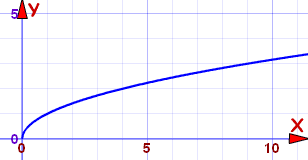# Square Root Function

This is the Square Root Function:

f(x) = √x

This is its graph:f(x) = √x

Its Domain is the Non-Negative Real Numbers: [0, +∞)

Its Range is also the Non-Negative Real Numbers: [0, +∞)

## As an Exponent

The Square Root Function can also be written as an exponent:

f(x) = x½

Plot the graph here